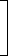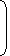# Tips and Tricks on Bank AccountIMPORTANT CONCEPTS

Banker’s Discount:

Suppose a merchant A buys goods worth, say Rs. 10,000 from another merchant B at a credit of say 5 months. Then, B prepares a bill, called the bill of exchange. A signs this bill and allows B to withdraw the amount from his bank account after exactly 5 months.

The date exactly after 5 months is called nominally due date. Three days (known as grace days) are added to it get a date, known as legally due date.

Suppose B wants to have the money before the legally due date. Then he can have the money from the banker or a broker, who deducts S.I. on the face vale (i.e., Rs. 10,000 in this case) for the period from the date on which the bill was discounted (i.e., paid by the banker) and the legally due date. This amount is know as Banker’s Discount (B.D.).

Thus, B.D. is the S.I. on the face value for the period from the date on which the bill was discounted and the legally due date.

Banker’s Gain (B.G.) = (B.D.) – (T.D.) for the unexpired time.

Note: When the date of the bill is not given, grace days are not to be added.

IMPORTANT FORMULAE

1.   B.D. = S.I. on bill for unexpired time.

 2.   B.G. = (B.D.) – (T.D.) = S.I. on T.D. = (T.D.)2 P.W.

3.   T.D. P.W. x B.G.

 4.   B.D. =Amount x Rate x Time100
 5.   T.D. =Amount x Rate x Time100 + (Rate x Time)
 6.   Amount =B.D. x T.D.B.D. – T.D.
 7.   T.D. =B.G. x 100Rate x Time
The banker’s discount of a certain sum of money is Rs. 72 and the true discount on the same sum for the same time is Rs. 60. The sum due is:
 A. Rs. 360 B. Rs. 432 C. Rs. 540 D. Rs. 1080

Explanation:

 Sum = B.D. x T.D. = Rs.72 x 60= Rs.72 x 60= Rs. 360. B.D. – T.D. 72 – 60 12
The certain worth of a certain sum due sometime hence is Rs. 1600 and the true discount is Rs. 160. The banker’s gain is:
 A. Rs. 20 B. Rs. 24 C. Rs. 16 D. Rs. 12

Explanation:

 B.G. = (T.D.)2 = Rs.160 x 160= Rs. 16. P.W. 1600

Correct! Wrong!

Correct! Wrong!

Correct! Wrong!

Correct! Wrong!

#### The banker's gain on a sum due 3 years hence at 12% per annum is Rs. 270. The banker's discount is:

Correct! Wrong!

QUIZ ON BANKER'S ACCOUNT
GREAT JOB!!!